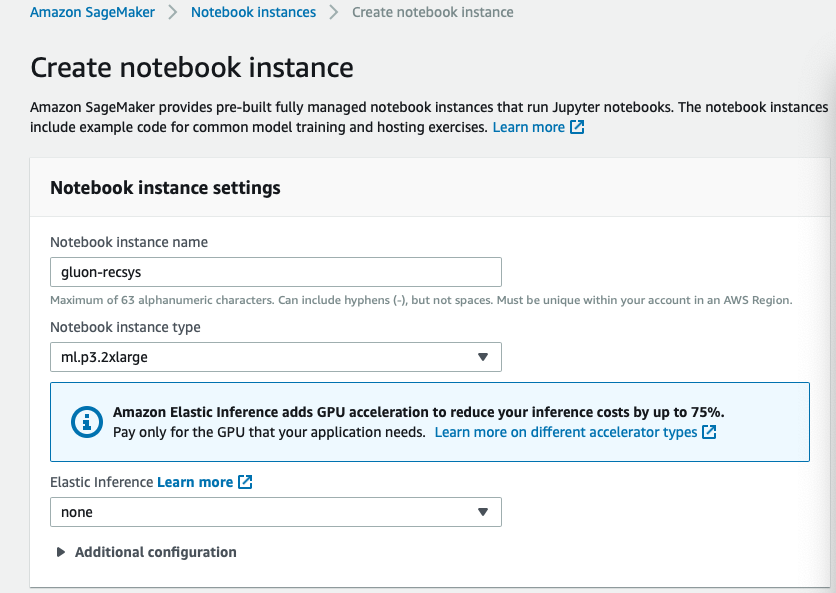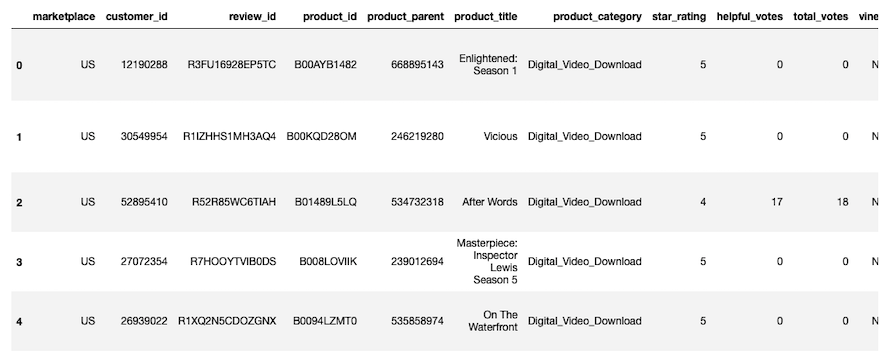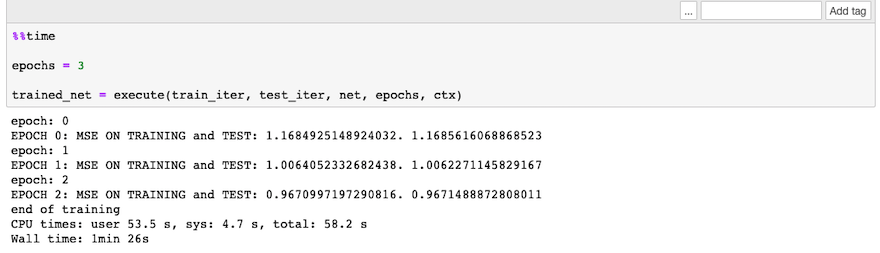# 使用 Amazon SageMaker 构建基于 gluon 的推荐系统

## 解决方案概览

1. 环境准备
2. 使用Jupyter Notebook下载数据集并将其进行数据预处理
3. 使用本地机器训练
4. 使用 Amazon SageMaker BYOS进行模型训练
5. 托管部署及推理测试

## 环境准备``````cd ~/SageMaker
git clone https://github.com/awslabs/amazon-sagemaker-examples/tree/master/introduction_to_applying_machine_learning/gluon_recommender_system
``````

## 使用 Jupyter Notebook 下载数据集并将其进行数据预处理

``````!mkdir /tmp/recsys/

## 数据预处理

``````df=pd.read_csv('/tmp/recsys/amazon_reviews_us_Digital_Video_Download_v1_00.tsv.gz', delimiter='\t',error_bad_lines=False)
``````• marketplace：两位数的国家编码，此处都是‘US’
• customer_id： 一个代表发表评论用户的随机编码，对于每个用户唯一
• review_id： 对于评论的唯一编码
• product_id： 亚马逊通用的产品编码
• product_parent：母产品编码，很多产品有同属于一个母产品
• product_title：产品的描述
• product_category：产品品类
• star_rating：评论星数，从1到5
• vine：是否为vine项目中的评论
• verified_purchase：该评论是否来源于已购买该产品的客户
• review_body：评论内容
• review_date：评论时间

``df = df[['customer_id', 'product_id', 'star_rating', 'product_title']]``

``````customers = df['customer_id'].value_counts()
products = df['product_id'].value_counts()
quantiles = [0, 0.01, 0.02, 0.03, 0.04, 0.05, 0.1, 0.25, 0.5, 0.75, 0.9, 0.95, 0.96, 0.97, 0.98, 0.99, 1]
print('customers\n', customers.quantile(quantiles))
print('products\n', products.quantile(quantiles))
``````

``````customers = customers[customers >= 5]
products = products[products >= 10]

reduced_df = df.merge(pd.DataFrame({'customer_id': customers.index})).merge(pd.DataFrame({'product_id': products.index}))
``````

``````customer_index = pd.DataFrame({'customer_id': customers.index, 'user': np.arange(customers.shape)})
product_index = pd.DataFrame({'product_id': products.index,
'item': np.arange(products.shape)})

reduced_df = reduced_df.merge(customer_index).merge(product_index)
``````

``````test_df = reduced_df.groupby('customer_id').last().reset_index()

train_df = reduced_df.merge(test_df[['customer_id', 'product_id']],
on=['customer_id', 'product_id'],
how='outer',
indicator=True)
train_df = train_df[(train_df['_merge'] == 'left_only')]
``````

``````batch_size = 1024

train = gluon.data.ArrayDataset(nd.array(train_df['user'].values, dtype=np.float32),
nd.array(train_df['item'].values, dtype=np.float32),
nd.array(train_df['star_rating'].values, dtype=np.float32))
test  = gluon.data.ArrayDataset(nd.array(test_df['user'].values, dtype=np.float32),
nd.array(test_df['item'].values, dtype=np.float32),
nd.array(test_df['star_rating'].values, dtype=np.float32))

train_iter = gluon.data.DataLoader(train, shuffle=True, num_workers=4, batch_size=batch_size, last_batch='rollover')
test_iter = gluon.data.DataLoader(train, shuffle=True, num_workers=4, batch_size=batch_size, last_batch='rollover')

``````

## 使用本地机器训练

• embeddings 将输入的稠密向量转换为固定长度。这里我们选取64，可调节
• dense layers 全链接层，使用ReLU激活函数
• dropout layer 用于防止过拟合
``````class MFBlock(gluon.HybridBlock):
def __init__(self, max_users, max_items, num_emb, dropout_p=0.5):
super(MFBlock, self).__init__()

self.max_users = max_users
self.max_items = max_items
self.dropout_p = dropout_p
self.num_emb = num_emb

with self.name_scope():
self.user_embeddings = gluon.nn.Embedding(max_users, num_emb)
self.item_embeddings = gluon.nn.Embedding(max_items, num_emb)

self.dropout_user = gluon.nn.Dropout(dropout_p)
self.dropout_item = gluon.nn.Dropout(dropout_p)

self.dense_user   = gluon.nn.Dense(num_emb, activation='relu')
self.dense_item = gluon.nn.Dense(num_emb, activation='relu')

def hybrid_forward(self, F, users, items):
a = self.user_embeddings(users)
a = self.dense_user(a)

b = self.item_embeddings(items)
b = self.dense_item(b)

predictions = self.dropout_user(a) * self.dropout_item(b)
predictions = F.sum(predictions, axis=1)
return predictions
##set up network
num_embeddings = 64
net = MFBlock(max_users=customer_index.shape,
max_items=product_index.shape,
num_emb=num_embeddings,
dropout_p=0.5)
# Initialize network parameters
ctx = mx.gpu()
net.collect_params().initialize(mx.init.Xavier(magnitude=60),
ctx=ctx,
force_reinit=True)
net.hybridize()

# Set optimization parameters
opt = 'sgd'
lr = 0.02
momentum = 0.9
wd = 0.

trainer = gluon.Trainer(net.collect_params(),
opt,
{'learning_rate': lr,
'wd': wd,
'momentum': momentum})
``````

``````def eval_net(data, net, ctx, loss_function):
acc = MSE()
for i, (user, item, label) in enumerate(data):

user = user.as_in_context(ctx)
item = item.as_in_context(ctx)
label = label.as_in_context(ctx)
predictions = net(user, item).reshape((batch_size, 1))
acc.update(preds=[predictions], labels=[label])

return acc.get()
``````

``````def execute(train_iter, test_iter, net, epochs, ctx):

loss_function = gluon.loss.L2Loss()
for e in range(epochs):

print("epoch: {}".format(e))

for i, (user, item, label) in enumerate(train_iter):
user = user.as_in_context(ctx)
item = item.as_in_context(ctx)
label = label.as_in_context(ctx)

output = net(user, item)
loss = loss_function(output, label)

loss.backward()
trainer.step(batch_size)

print("EPOCH {}: MSE ON TRAINING and TEST: {}. {}".format(e,
eval_net(train_iter, net, ctx, loss_function),
eval_net(test_iter, net, ctx, loss_function)))
print("end of training")
return net
%%time
epochs = 3
trained_net = execute(train_iter, test_iter, net, epochs, ctx)
``````## 使用 Amazon SageMaker BYOS进行模型训练

• 将所有数据预处理的工作封装至一个函数，本文中为prepare_train_data
• 将所有训练相关的代码（函数或类）复制粘贴
• 定义一个名为train的函数，用于：
• 添加一段Sagemaker训练集群读取数据的代码
• 定义超参数为一个字典作为入参，在之前的例子我们是全局定义的
• 创建网络并且执行训练

``````boto3.client('s3').copy({'Bucket': 'amazon-reviews-pds',
bucket,
``````

• 训练的实例类型和实例个数，SageMaker提供的MXNet容器支持单机训练也支持多gpu训练，只需要您指定训练机型个数即可切换
• 模型存储的S3路径及其对应的权限设置
• 模型对应的超参数，这里我们将embedding的个数提升，后面可以看到这个结果会优于之前的结果，这里的超参数配置可以进一步进行调优，从而得到更为准确的模型

``````m = MXNet('recommender.py',
py_version='py3',
role=role,
train_instance_count=1,
train_instance_type="ml.p2.xlarge",
output_path='s3://{}/{}/output'.format(bucket, prefix),
hyperparameters={'num_embeddings': 512,
'opt': opt,
'lr': lr,
'momentum': momentum,
'wd': wd,
'epochs': 10},
framework_version='1.1')

m.fit({'train': 's3://{}/{}/train/'.format(bucket, prefix)})
``````

## 托管部署及推理测试

``````predictor = m.deploy(initial_instance_count=1,
instance_type='ml.m4.xlarge')
predictor.serializer = None
``````

``````predictor.predict(json.dumps({'customer_id': customer_index[customer_index['user'] == 6]['customer_id'].values.tolist(),
'product_id': ['B00KH1O9HW', 'B00M5KODWO']}))``````

``````test_preds = []
for array in np.array_split(test_df[['customer_id', 'product_id']].values, 40):
test_preds += predictor.predict(json.dumps({'customer_id': array[:, 0].tolist(),
'product_id': array[:, 1].tolist()}))

test_preds = np.array(test_preds)
print('MSE:', np.mean((test_df['star_rating'] - test_preds) ** 2))
``````

## 参考资料

1. 本文代码： https://github.com/awslabs/amazon-sagemaker-examples/tree/master/introduction_to_applying_machine_learning/gluon_recommender_system
2. Apriori算法： https://en.wikipedia.org/wiki/Apriori_algorithm
3. MXNet： https://mxnet.incubator.apache.org
4. Amazon SageMaker: https://docs.aws.amazon.com/sagemaker/index.html
5. 动手深度学习： http://zh.d2l.ai/chapter_preface/preface.html

## 本篇作者TAGS: